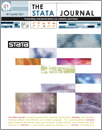Home  >>  Archives  >>  Volume 11 Number 3  >>  st0235

#### The Stata JournalVolume 11 Number 3: pp. 403-419

Subscribe to the Stata Journal## A closer examination of three small-sample approximations to the multiple-imputation degrees of freedom

 David A. Wagstaff HHD Consulting Group College of Health and Human Development Pennsylvania State University daw22@psu.edu Ofer Harel Department of Statistics University of Connecticut
Abstract.  Incomplete data is a common complication in applied research. In this study, we use simulation to compare two approaches to the multiple imputation of a continuous predictor: multiple imputation through chained equations and multivariate normal imputation. This study extends earlier work by being the first to 1) compare the small-sample approximations to the multiple-imputation degrees of freedom proposed by Barnard and Rubin (1999, Biometrika 86: 948–955); Lipsitz, Parzen, and Zhao (2002, Journal of Statistical Computation and Simulation 72: 309–318); and Reiter (2007, Biometrika 94: 502–508) and 2) ask if the sampling distribution of the t statistics is in fact a Student’s t distribution with the specified degrees of freedom.

In addition to varying the imputation method, we varied the number of imputations (m = 5, 10, 20, 100) that were averaged over 500,000 replications to obtain the combined estimates and standard errors for a linear model that regressed the log price of a home on its age (years) and size (square feet) in a sample of 25 observations. Six age values were randomly set equal to missing for each replication.

As assessed by the absolute percentage and relative percentage bias, the two approaches performed similarly. The absolute bias of the regression coefficients for age and size was roughly −0.1% across the levels of m for both approaches; the absolute bias for the constant was 0.6% for the chained-equations approach and 1.0% for the multivariate normal model. The absolute biases of the standard errors for age, size, and the constant were 0.2%, 0.3%, and 1.2%, respectively. In general, the relative percentage bias was slightly smaller for the chained-equations approach. Graphical and numerical inspection of the empirical sampling distributions for the three t statistics suggested that the area from the shoulder to the tail was reasonably well approximated by a t distribution and that the small-sample approximations to the multiple-imputation degrees of freedom proposed by Barnard and Rubin and by Reiter performed satisfactorily.

View all articles by these authors: David A. Wagstaff, Ofer Harel

View all articles with these keywords: missing data, multiple imputation, small-sample degrees of freedom